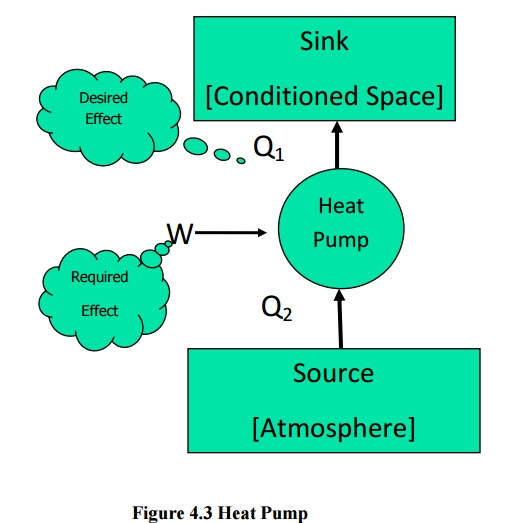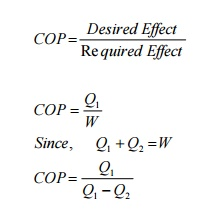Home | | Engineering Thermodynamics | The Second law of Thermodynamics

# The Second law of Thermodynamics

The term thermal reservoir refers to a very large system in stable equilibrium, to which or from which, any amount of heat can be transferred at constant temperature.

The Second law of Thermodynamics

Kelvin Planck’s: It is impossible statement to constructa device that, operating continuously, will produce no effect other than transfer of heat from a single thermal reservoir and performance of an equal amount of work.

The term thermal reservoir refers to a very large system in stable equilibrium, to which or from which, any amount of heat can be transferred at constant temperature.

A thermal reservoir supplying heat continuously at constant temperature is known as source. (Example : Sun)

A thermal reservoir receiving heat continuously at constant temperature is known as sink. (Examples : River, Sea)

From Kelvin-Planck statement it is clear that for any system to operate in a cycle and to give out work continuously it should interact with a minimum of two reservoirs at different temperatures. The system will receive heat from the high temperature reservoir and reject heat to the low temperature reservoir. Such devices are known as heat engines. Performance (or) Efficiency of a heat engine can be expressed as the ratio of desired output to the required input. In a heat engine the desired output is net work output and the required input is total heat inputFrom first law of thermodynamicsClausius statement : Unaided by an external agency heat can not be transferred from a body at lower temperature to a body at higher temperature.

Devices that are used to transfer heat from a body at lower temperature to a body at higher temperature are known as refrigerators (or) heat pumps. If the high temperature side is atmosphere it is a refrigerator. If the low temperature side is atmosphere it is known as a heat pump. The performance index here is called coefficient of performance (COP). In refrigerator (and heat pumps) the performance is the ratio of two independent parameters and hence the possibility of getting the value more than unity is always there. But the term efficiency is restricted to a maximum of unity. Hence the term efficiency is not used here.COP=Q/W

Taking work as external agency, for refrigerators (Figure 4.2)

From first law

QS=  SW

Q1 -Q2  =W

COP = Q2 / Q1Q2Figure 4.2 RefrigeratorSimilarly for a heat pumps (Figure 4.3)Study Material, Lecturing Notes, Assignment, Reference, Wiki description explanation, brief detail
Mechanical : Engineering Thermodynamics : The Second Law of Thermodynamics : The Second law of Thermodynamics |